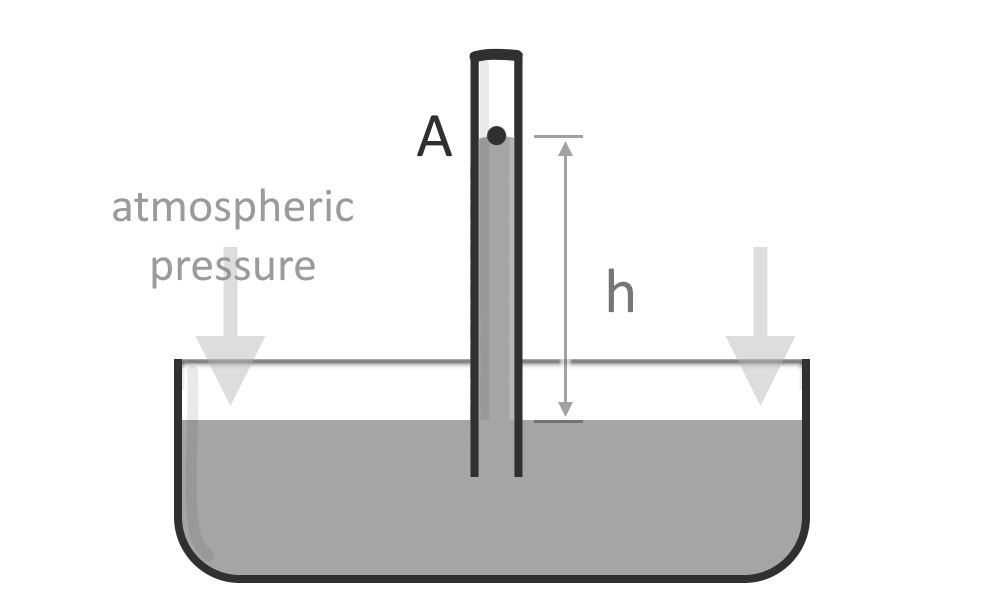# Manometer Calculator

Created by Krishna Nelaturu
Last updated: Nov 05, 2022

With our manometer calculator, you can calculate the pressure in a fluid flow using the height of the liquid in a manometer. In this article, we shall discuss what a manometer is and how to calculate the pressure in a manometer. So grab your favorite drink, and let's set off to demystify this liquid in a bent glass tube as we peek at the manometry equation!

If you want to calculate pressure using the force applied, head to our pressure calculator instead.

## What is a manometer?

A manometer is an instrument that can measure pressure in gases, liquids, or solids. A manometer measures the force exerted by a fluid on a surface. This pressure is then converted into a readable format, allowing users to observe and compare pressure readings easily. Manometers are often used in laboratory settings to calibrate other instruments. Their usage in meteorology to measure atmospheric pressure is no secret to anyone! We can also use them to diagnose pressure problems in systems such as pipelines and combustion engines.

The mercury manometer is the most common type, which uses mercury as the liquid. The mercury is placed in a U-shaped tube, with one end open to the air and the other connected to the location (say, a fluid pipe) where we want to measure the pressure. As the pressure in the area increases, it forces the column of mercury upwards, and vice-versa. At any point, the hydrostatic pressure in the liquid column will be in equilibrium with the pressure in the area.

We can read the mercury level in the tube and determine the pressure in the area. This last step, of course, requires using a manometry equation to calculate the pressure from the height of the liquid.

Other manometers use different liquids, such as water or oil, but they all work on the same principle. Using a liquid column to measure pressure, manometers can provide a more accurate reading than other pressure-measuring devices.

## Manometer pressure formula

A manometer reading will tell us the height of the liquid column only. We need to use the manometer pressure formula to calculate the pressure in a system. This formula is:

$P = \rho \cdot g \cdot h$

Where

• $P$ - The pressure in the system (gauge pressure or manometer pressure);
• $\rho$ - The density of the liquid in the manometer;
• $g$ - The gravitational acceleration (approximately $9.81 \rm{m/s^2}$); and
• $h$ - The height of the liquid column.

As you can see, the pressure calculation is a simple matter of multiplication - Multiply the liquid column height by the liquid's density and the gravitational acceleration. It gets slightly more complex when multiple fluids are involved, but that's where our manometer calculator comes in!

Also, if you want to know more about different formulae in fluid mechanics, visit our Bernoulli equation calculator.

## Using this manometer calculator

We built this manometer calculator to be as versatile as possible, enabling you to skip complicated calculations in different scenarios. Here are the different cases it can handle:

1. Single column manometer
• Manometer in an open tank.
• Manometer attached to a tank or a pipe.
2. U-tube manometer open to the air on both ends
• Only one fluid in the manometer.
• Two different fluids in the manometer.
3. U-tube manometer attached to a pipe on one end.
4. U-tube manometer attached to two different pipes on its two ends.

Simply choose which scenario describes your case best. Then proceed to provide all the relevant information, including:

• Density of the fluids.
• Height of the fluids.
• Whether point B is higher than A.
• In the advanced mode of this manometer calculator, you can also change the gravitational acceleration value.
Krishna Nelaturu
Manometer type
single-column
Manometer is attached to...
an open tankAtmospheric pressure
lbs/ft²
Density, ρ
lb/cu ft
Height of liquid, h
in
Gauge pressure at point A
lbs/ft²
Absolute pressure at point A
lbs/ft²
People also viewed…

### Drag equation

Learn how to calculate the equation for the drag force in the blink of an eye: from the formula to the drag coefficient, we will cover all your doubts!

### Lift coefficient

Use this lift coefficient calculator to determine the coefficient of lift for your wing design.